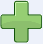# Algebra - Substitution

"Substitute" means to put in the place of another.

## Substitution

In Algebra "Substitution" means putting numbers where the letters are:When we have:
 x − 2And we know that x=6 ...... then we can "substitute" 6 for x:
 6 − 2 = 4

### Example: When x=2, what is 10/x + 4 ?

Put "2" where "x" is:

10/2 + 4 = 5 + 4 = 9### Example: When x=5, what is x + x/2 ?

Put "5" where "x" is:

5 + 5/2 = 5 + 2.5 = 7.5

### Example: If x=3 and y=4, then what is x2 + xy ?

Put "3" where "x" is, and "4" where "y" is:

32 + 3×4 = 3×3 + 12 = 21

### Example: If x=3 (but we don't know "y"), then what is x2 + xy ?

Put "3" where "x" is:

32 + 3y = 9 + 3y

(that is as far as we can get)

As that last example showed, we may not always get a number for an answer, sometimes just a simpler formula.

## Negative Numbers

When substituting negative numbers, put () around them so you get the calculations right.

### Example: If x = −2, then what is 1 − x + x2 ?

Put "(−2)" where "x" is:

1 − (−2) + (−2)2 = 1 + 2 + 4 = 7

In that last example:

• the − (−2) became +2
• the (−2)2 became +4

because of these special rules:

Rule Adding or   Multiplying or Subtracting DividingTwo like signs become a positive sign 3+(+2) = 3 + 2 = 5 3 × 2 = 6 6−(−3) = 6 + 3 = 9 (−3) × (−2) = 6Two unlike signs become a negative sign 7+(−2) = 7 − 2 = 5 3 × (−2) = −6 8−(+2) = 8 − 2 = 6 (−3) × 2 = −6